# Chezy’s Constant and Manning’s Coefficient for Open ChannelsReading time: 1 minute

OBJECTIVE: i. To find Chezy’s constant for open channels. ii. To find Manning’s Roughness coefficient for the given open channel. iii. To draw the following graphs for two different slopes of channel. iv. Chezy’s constant C vs. Reynolds number. v. Manning’s coefficient n vs. Reynolds number. EQUIPMENT: a) Open channel of rectangular cross section with slope adjusting mechanism. b) Pointer gauge. c) Measuring tank. d) Stop watch . BASICS: Allow water to flow uniformly through the given open channel of slop s at different rates. The actual discharge Qa is determined by using measuring tank and the stop watch. The actual discharge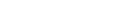Where a -area of measuring tank in cm2. h -Level difference of water in the measuring tank in cm. tm-The mean time to collect water for a height difference of h cm, measured in sec Chezy’s constant C for open channels can be calculated from the relationship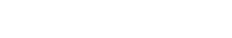Where A -B*H cm2 ( B-width of the channel-depth of water) S -tan (slope of channel bed) Rh -Hydraulic radius (Cross sectional are/ Wetted perimeter) (B*H) /(2H+B) CM Manning’s roughness coefficient n can be calculated using the formula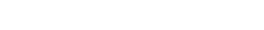Where A, Rh , and s are stated above. Reynolds number for flow through the pipe line is calculated as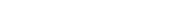Where ? -Mass density of water(995.7 )kg / m 3 v -Velocity of flow cm/s. Rh -Hydraulic radius of channel in cm µ - Absolute viscosity of water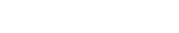OBSERVATIONS: Constants i. Measuring tank size ii. The height h cm for which the time t1 and t2 are noted to collect water in the measuring tank iii. Width of the channel B cm iv. Slope s of the channel tan? =y/L (L-test length horizontal, y-vertical shift of channel shown by the pointer) v. Temperature of water flowing through the pipe line Variables i. The depth of water H cm in the channel measured by pointer gauge ii. Time tm seconds required to collect water for a height of h cm in the measuring tank as mean value of readings t1 and t2.PROCEDURE: i. Set the channel for the required slope and allow water to flow through the channel ii. Adjust the inlet valve to get the maximum depth of water flow in the channel and measure the depth as H cm, using pointer gauge .Divide the value in to seven approximately equal divisions to fix the steps in depth for the seven sets of readings iii. Adjust the inlet valve opening to get the required depth of water in the channel iv. Note the time t to collect water for a rise of h cm in the measuring tank twice as t1 and t2.If the differences in reading exceeds 10% take a third reading which is coming in the range v. Repeat the experiment for different depths of water vi. Do the above experiment again for a different slope and tabulate all the observations RESULT: INFERENCE: